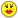+0

# A 5-pack of jump ropes costs \$15.15. What is the unit price?

+3
462
1

A 5-pack of jump ropes costs \$15.15. What is the unit price?

#1
+8

I'm going to let you try to answer that question. You can do it!I'll give you an example just like that one ok

If Brand X costs \$3.99 for 32 oz, let's figure out the unit cost of Brand X (the cost of 1 oz).

It's easy!!!

Divide \$3.99 by 32 and round to the nearest tenth
(the cost goes inside the division box).

The unit cost is approximately \$0.12.

welll lets see if you can do it

HAVE A NICE DAY!Nov 25, 2014

#1
+8

I'm going to let you try to answer that question. You can do it!I'll give you an example just like that one ok

If Brand X costs \$3.99 for 32 oz, let's figure out the unit cost of Brand X (the cost of 1 oz).

It's easy!!!

Divide \$3.99 by 32 and round to the nearest tenth
(the cost goes inside the division box).

The unit cost is approximately \$0.12.

welll lets see if you can do it

HAVE A NICE DAY!SelenaPubblT Nov 25, 2014